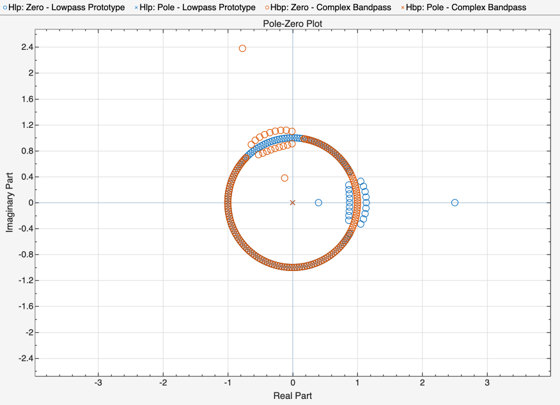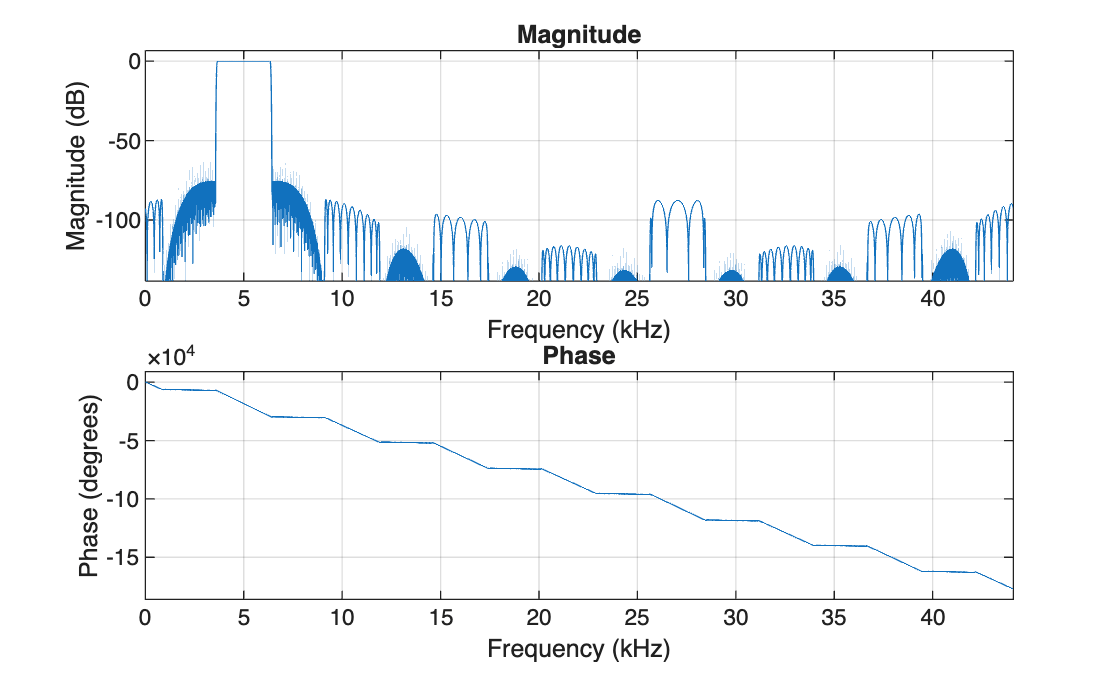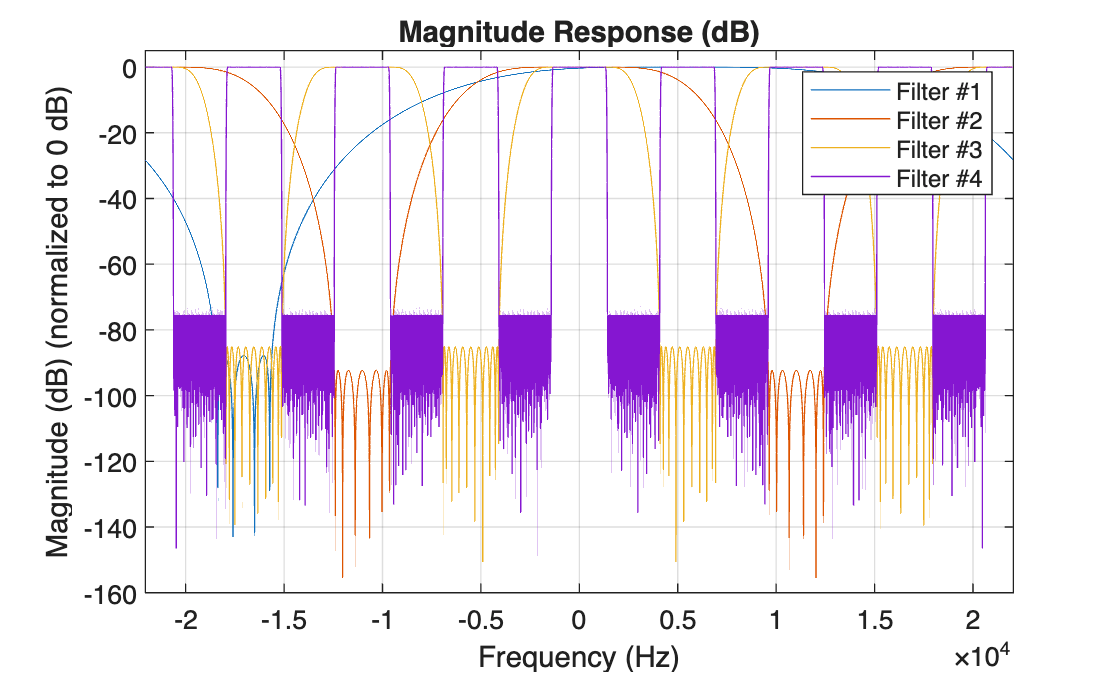# Complex Bandpass Filter Design

This example shows how to design complex bandpass filters. Complex bandpass filters are used in many applications from IF subsampling digital down converters to vestigial sideband modulation schemes for analog and digital television broadcast. One easy way to design a complex bandpass filter is to start with a lowpass prototype and apply a complex shift frequency transformation. In this example, we review several cases of lowpass prototypes from single-stage single-rate FIR filters to multistage multirate FIR filters to IIR filters.

### Single-Stage Single-Rate FIR Design

In the case of a single-rate FIR design, we simply multiply each set of coefficients by (aka 'heterodyne with') a complex exponential. In the next example, we rotate the zeros of the lowpass Nyquist filter prototype by a normalized frequency of .6.

```Hlp = design(fdesign.nyquist(8), SystemObject=true); % Lowpass prototype N = length(Hlp.Numerator)-1; Fc = .6; % Desired frequency shift Hbp = clone(Hlp); Hbp.Numerator = Hbp.Numerator.*exp(1j*Fc*pi*(0:N)); hfvt = fvtool(Hlp,Hbp,Color='white'); legend(hfvt,'Lowpass Prototype','Complex Bandpass',Location='NorthWest')```The same technique also applies to single-stage multirate filters.

### Multirate Multistage FIR Design

In the case of multirate multistage FIR filters, we need to account for the different relative frequencies each filter operates on. In the case of a multistage decimator, the desired frequency shift applies only to the first stage. Subsequent stages must also scale the desired frequency shift by their respective cumulative decimation factor.

```Hd = designMultistageDecimator(16,16,0.1,75); Fc = -.2; % Desired frequency shift Hdbp = clone(Hd); Fck = Fc; for k = 1:Hdbp.getNumStages Stagek = Hdbp.(sprintf('Stage%i',k)); Nk = length(Stagek.Numerator)-1; Stagek.Numerator = Stagek.Numerator.*exp(1j*Fck*pi*(0:Nk)); % Update the frequency shift applied to the k-th stage Fck = Fck* Stagek.DecimationFactor; end hfvt = fvtool(Hd,Hdbp); legend(hfvt,'Lowpass Prototype','Complex Bandpass',Location='NorthWest')```Similarly, in the case of a multistage interpolator, the desired frequency shift applies only to the last stage. Previous stages must also scale the desired frequency shift by their respective cumulative interpolation factor.

```Hi = designMultistageInterpolator(16,16,0.1,75); Fc = .4; % Desired frequency shift Hibp = clone(Hi); Fck = Fc; for k = Hibp.getNumStages:-1:1 Stagek = Hibp.(sprintf('Stage%i',k)); Nk = length(Stagek.Numerator)-1; Stagek.Numerator = Stagek.Numerator.*exp(1j*Fck*pi*(0:Nk)); % Update the frequency shift applied to the k-th stage Fck = Fck* Stagek.InterpolationFactor; end hfvt = fvtool(Hi,Hibp); legend(hfvt,'Lowpass Prototype','Complex Bandpass',Location='NorthWest')```We can design multistage bandpass filters easily by using the `dsp.ComplexBandpassDecimator` System object. The object designs the bandpass filter based on the specified decimation factor, center frequency, and sample rate. There is no need to translate lowpass coefficients to bandpass as we did in the section above: the object will do it for us.

Design a complex bandpass filter with a decimation factor of 16, a center frequency of 5 KHz, a sampling rate of 44.1 KHz, a transition width of 100 Hz, and a stopband attenuation of 75 dB:

```bp = dsp.ComplexBandpassDecimator(16,5000,SampleRate=44100,... TransitionWidth=100,... StopbandAttenuation=75);```

Visualize the filter response using `freqz`:

`freqz(bp)`Visualize the response of the different filter stages using `visualizeFilterStages`:

`visualizeFilterStages(bp);`Notice that only the first filter is shifted to 5 KHz. The subsequent filter stages are lowpass and have real coefficients. Set the `MinimizeComplexCoefficients` property to false to shift all filter stages to 5000 KHz.

Get the cost of the bandpass filter using `cost`:

`cost(bp)`
```ans = struct with fields: NumCoefficients: 144 NumStates: 272 RealMultiplicationsPerInputSample: 27.8750 RealAdditionsPerInputSample: 27 ```

### Single-Rate IIR Design

Finally in case of single-rate IIR designs, we can either use a complex shift frequency transformation or a lowpass to complex bandpass IIR transformation. In the latter case, the bandwidth of the bandpass filter may also be modified.

```Fp = .2; % Design a lowpass prototype, and obtain the second order coefficients Hiirlp = design(fdesign.lowpass(Fp,.25,.5,80),'ellip', SystemObject=true); B = Hiirlp.ScaleValues(1:end-1).*Hiirlp.SOSMatrix(:,1:3); A = Hiirlp.SOSMatrix(:,4:6); % Perform lowpass to complex bandpass transform Fc = .6; % Desired frequency shift [Bc,Ac] = iirlp2bpc(B,A, ... % Transform lowpass to complex bandpass Fp, [Fc-Fp, Fc+Fp]); % Lowpass passband frequency mapped % to bandpass passband frequencies % Construct a filter object and plot the responses Hiircbp = dsp.SOSFilter(Bc, Ac); hfvt = fvtool(Hiirlp,Hiircbp); legend(hfvt,'Lowpass Prototype', 'Complex Bandpass',Location='NorthWest')```